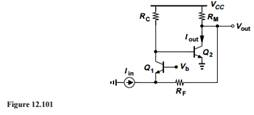### Create an Account

Home / Questions / Consider the feedback circuit depicted in Fig 12101 Assume VA 1 a Suppose the output qua...

# Consider the feedback circuit depicted in Fig 12101 Assume VA 1 a Suppose the output quantity of interest is the collector current of Q2, Iout Assuming RM is very small and RF is very large,

Consider the feedback circuit depicted in Fig. 12.101. Assume VA = 1.

(a) Suppose the output quantity of interest is the collector current of Q2, Iout. Assuming Ris very small and RF is very large, determine the closed-loop gain and I/O impedances of the circuit.

(b) Now, suppose the output quantity of interest is Vout. Assuming RF is very large, compute the closed-loop gain and I/O impedances of the circuit.Apr 24 2020 View more View LessSubscribe To Get Solution